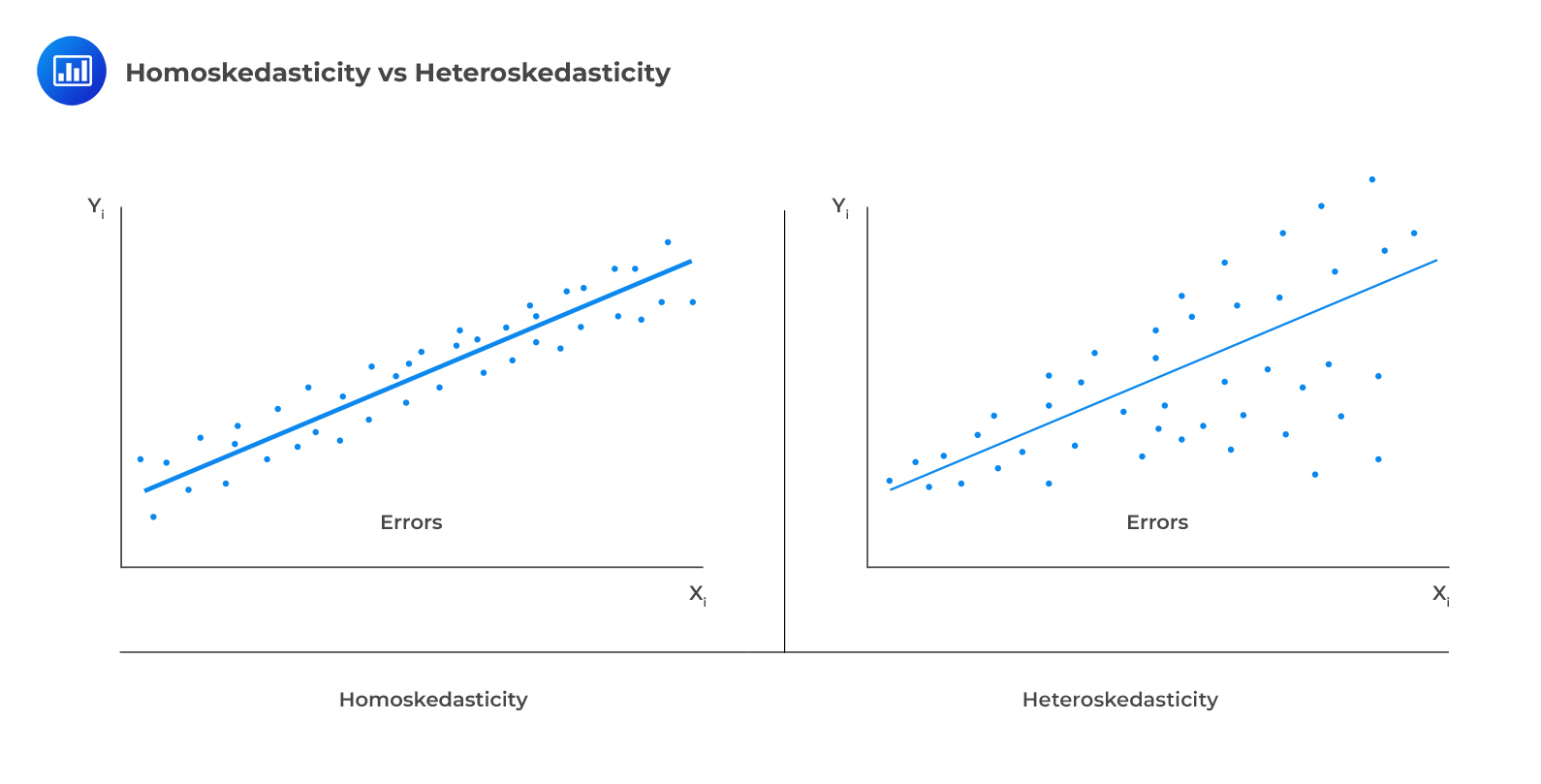# Autoregressive Conditional Heteroskedasticity

Heteroskedasticity is the dependence of the variance of the error term on the independent variable. We have been assuming that time series follows the homoskedasticity assumption. Homoskedasticity is the independence of the variance of the error term on the independent variable. Violation of this condition leads to heteroskedasticity.Heteroskedasticity in the autoregressive model makes the standard errors of the regression coefficients of the model invalid, leading to misleading interpretations.

## Autoregressive Conditional Heteroskedasticity (ARCH) Models

Autoregressive conditional heteroskedasticity is a problem associated with the correlation of variances of the error terms. An ARCH(1) model is an AR(1) model with conditional heteroskedasticity.

The error terms in an ARCH(1) model are normally distributed with a mean of 0 and a variance of $$\text{a}_{0}+\text{a}_{1}\epsilon_{\text{t}-1}^{2}$$.

$$\epsilon_{\text{t}}∼\text{N}(0, \text{a}_{0}+\text{a}_{1}\epsilon_{\text{t}-1}^{2})$$

Steps for Testing for ARCH(1) Conditional Heteroskedasticity

1. Regress the squared residuals from each period on the prior period squared residuals.
2. Estimate $$\hat{\epsilon}_{\text{t}}^{2}=\alpha_{0}+\alpha_{1}\hat{\epsilon}_{\text{t}-1}^{2}+\mu_{\text{t}}$$.
3. If the estimated slope coefficient  $$\hat{\alpha}_1$$ is statistically different from 0, the series shows an ARCH(1) effect, and thus the need to correct for heteroskedasticity.
4. We can then predict the variance of the errors for time $$t+1$$.

## Predicting Variance

Given an ARCH(1) model, we can use the parameter estimates from our test for conditional heteroskedasticity to predict next period variance as:

$$\hat{\sigma}_{\text{t}+1}^{2}=\hat{\alpha}_{0}+\hat{\alpha}_{1}\hat{\epsilon}_{\text{t}}^{2}$$

#### Example: Testing for ARCH (1) and Predicting the Variance of Errors

The following results have been obtained from regressing the squared residuals of an estimated autoregressive model on a constant and one lag of the squared error terms.

###### Regression Statistic

$$\small{\begin{array}{c|c} \text{R-squared} & 0.0430 \\ \hline\text{Standard error} & 24.638 \\ \hline\text{Observations} & 358 \\ \text{Durbin-Watson} & 2.1021\\ \hline\end{array}}$$

$$\small{\begin{array}{c|c|c|c} {}&\textbf{Coefficient}&\textbf{Standard Error}&\textbf{t-Statistic}\\ \hline\text{Intercept}&9.1680&1.4104&6.55002\\ \hline \hat{\epsilon}_{\text{t}-1}^{2}&0.2050&0.0506&4.0514\\ \end{array}}$$

1. Test whether the original time series has ARCH(1) errors at the 5% significance level.
2. If the squared error in the current period is 0.5%, predicted variance in the next period is closest to:

Solution

The test involves the first lag of the residuals of the approximated time series model, and thus the number of the observations is less by one. The number of degrees of freedom is $$358-1=357$$, and the corresponding critical t-value at the 5% level of significance is 1.97.

The t-statistic on $$\hat{\epsilon}_{\text{t}-1}^{2}$$ is greater than the critical value. This implies that $$\hat{\alpha}_{1}$$ is statistically significantly different from 0. Therefore, the time series has ARCH(1) errors.

The predicted variance for the next period is determined using the formula:

$$\hat{\sigma}_{\text{t}}^{2}=\hat{\alpha}_{0}+\hat{\alpha}_{1}\hat{\epsilon}_{\text{t}-1}^{2}$$

$$\hat{\sigma}_{\text{t}}^{2}=9.1680+0.2050\hat{\epsilon}_{\text{t}-1}^{2}$$

So that the variance for the next period, in this case, is:

$$\hat{\sigma}_{\text{t}}^{2}=9.1680+0.2050\times0.005=9.169025$$

## Question

Consider the following regression results for an ARCH(1) model:

$$\small{\begin{array}{c|c|c} {}&\textbf{Coefficients} & \textbf{P-value} \\ \hline\text{Constant} & 4.8347 & <0.001 \\ \hline\text{Lag 1} & 0.2678 & <0.001\\ \end{array}}$$

Given that the current period squared error is 0.678, the predicted variance of the error terms in the next period is closest to:

1. 4.835.
2. 5.103.
3. 5.016.

#### Solution

The predicted variance for the next period is determined using the formula:

$$\hat{\sigma}_{\text{t}}^{2}=\hat{\alpha}_{0}+\hat{\alpha}_{1}\hat{\epsilon}_{\text{t}-1}^{2}$$

$$\hat{\sigma}_{\text{t}}^{2}=4.8347+0.2678\times0.678=5.016$$

LOS 5 (m) Explain autoregressive conditional heteroskedasticity (ARCH) and describe how ARCH models can be applied to predict the variance of a time series.

Shop CFA® Exam Prep

Offered by AnalystPrepLevel I
Level II
Level III
All Three Levels
Featured Shop FRM® Exam PrepFRM Part I
FRM Part II
FRM Part I & Part II
Learn with Us

Subscribe to our newsletter and keep up with the latest and greatest tips for success
Shop Actuarial Exams PrepExam P (Probability)
Exam FM (Financial Mathematics)
Exams P & FMGMAT® Complete CourseDaniel Glyn
2021-03-24
I have finished my FRM1 thanks to AnalystPrep. And now using AnalystPrep for my FRM2 preparation. Professor Forjan is brilliant. He gives such good explanations and analogies. And more than anything makes learning fun. A big thank you to Analystprep and Professor Forjan. 5 stars all the way!michael walshe
2021-03-18
Professor James' videos are excellent for understanding the underlying theories behind financial engineering / financial analysis. The AnalystPrep videos were better than any of the others that I searched through on YouTube for providing a clear explanation of some concepts, such as Portfolio theory, CAPM, and Arbitrage Pricing theory. Watching these cleared up many of the unclarities I had in my head. Highly recommended.Nyka Smith
2021-02-18
Every concept is very well explained by Nilay Arun. kudos to you man!2021-02-13